Students / SubjectsHandbook > Consumer Theory > Choice and Preferences > Representation of PreferencesPrinter Friendly

Representation of Preferences

A consumer's preferences can be represented by a utility function if they satisfy properties P.1 through P.4, and one additional property called continuity. Continuity is probably the least intuitive property of preferences, yet it is not implausible.

P.5 The "Continuity" Property

Preferences are continuous if the set of all choices that are at least as good as a choice x' and the set of all choices that are no better than x' are both closed sets. In the notation of sets, this is written as {x : x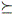x'} and {x : x'x} are both closed.

One definition of a closed set is that any sequence of points in the set that converges, converges to a point of the set. In this context, that means that for a sequence of points {xn} with n = 1, 2, 3, ..., if xxn for every xn and if xn converges to some consumption point x', then xx'.

Figure 6 shows an example of this. In the figure, if xxn for every n, and if xn converges to x', then continuity implies that xx'.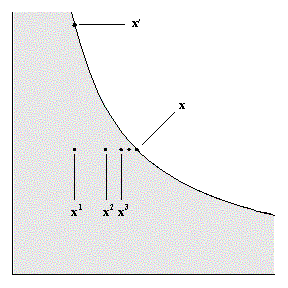Figure 6: Sequence of points xn that converge to x'.

Representation Theorem

If a consumer has a preference relation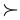that is complete, reflexive, transitive, strongly monotonic, and continuous, then these preferences can be represented by a continuous utility function u(x) such that u(x) > u(x') if and only if xx'.

Proof : Let e = (1, 1, ..., 1). For each x, define u(x) by u(x) e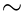x. Then u(x) is a utility function for the preferencesif
1. such a function u(x) exists,
2. the function u(x) is unique, and
3. u(x) > u(x') if and only if xx'.

Let B = {a: a ex}. If x = (x1, x2), let y = (max{x1, x2}, max{x1, x2}). Then strong monotonicity implies that yx, so B is not empty. Let W = {a: xa e}. Then 0 is an element of W, so W is not empty. By completeness, B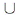W = {a : a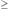0}. Both B and W are closed sets, from property P.5 (continuity), so B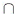W is not empty. Therefore, there is some a such that a ex. By strong monotonicity, if a' > a, then a' ex, and if a' < a, then xa' e, so a is unique. Let u(x) = a. So u(x) exists and it is unique.

Next, we want to show that u(x) represents the preferences. Suppose that x and y are two consumption levels and u(x) = ax where a x ex. Let u(y) = a y where a y ey. If a x > a y then by monotonicty a x ea y e. By transitivity, xa x ea y ey. Finally, if xy, then a x ea y e so that a x > a y.

Next